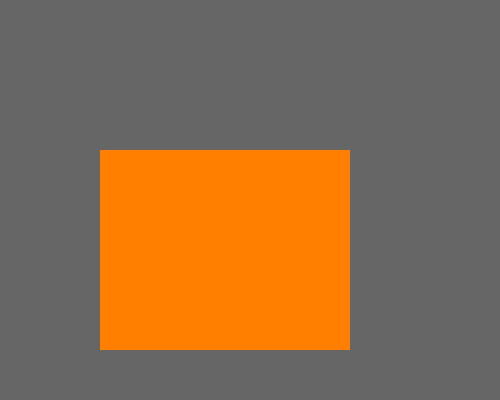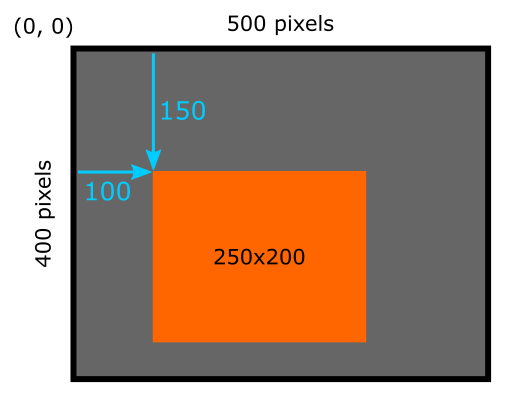# Simple image in generativepy

Martin McBride, 2020-08-29
Tags generativepy tutorial rectangle
Categories generativepy generativepy tutorial

In this article we will create a very simple image using generativepy. The image looks like this:## The code

Here is the code to draw the image above:

from generativepy.drawing import make_image, setup
from generativepy.color import Color
from generativepy.geometry import Rectangle

def draw(ctx, width, height, frame_no, frame_count):
setup(ctx, width, height, background=Color(0.4))
color = Color(1, 0.5, 0)
Rectangle(ctx).of_corner_size((100, 150), 250, 200).fill(color)

make_image("rectangle.png", draw, 500, 400)


We will now look at this in more detail.

## make_image

The make_image function, from the drawing module, is the main function that handles creating the image.

The basic parameters are:

• The filename of the output PNG file.
• A draw function that does the actual drawing.
• The width and height of the image in pixels.

The draw function is a function that we define ourselves to perform the drawing. It doesn't have to be called draw, of course, but it must have the correct number of parameters.

## draw function

draw accepts 5 parameters:

• ctx is a Pycairo context. This is a like a virtual drawing surface that you can draw on in code. Whatever you draw will appear on the final image.
• width and height are the width and height of the image in pixels. These are the values that we passed into make_image.
• frame_no and frame_count are only used when you want to create image sequences (for videos), so we can ignore them here.

generativepy uses Pycairo as its drawing library. Pycairo has an extensive set of drawing functions that you can use to draw on the context, but generativepy also provides some extra functions that are useful for generative art. You can mix and match these functions - that is especially useful if you want to use some advanced feature of Pycairo that generativepy doesn't directly support.

Our draw function calls the setup function to set up the drawing area, then draws a rectangle.

## setup function

The setup function isn't mandatory, but it does some useful things so you will often want to call it.

setup accepts 3 required parameters - the ctx, width and height that were passed in to the draw function. It has some optional parameters tat do various things.

In this case we are setting the background parameter to Color(0.4) which sets the background colour to a mid grey (it sets r, g and b to 40%).

We also create another colour, Color(1, 0.5, 0) for use later on. This is 100% red, 50% green and 0% blue, which is an orange colour.

## Drawing a rectangle

We use a Rectangle object to draw a rectangle, like this:

Rectangle(ctx).of_corner_size((100, 150), 250, 200).fill(color)


All shapes are drawn using the same pattern:

• Declare a shape object, for example Rectangle(ctx). The context is passed in at this stage.
• An of_xxx function is used to define the shape. In this case of_corner_size defines a rectangle from the position of its corner, and its width and height. The corner is specified using an (x, y) tuple.
• A drawing function is then used to actually draw the shape. In this case we use fill that fills the shape with the supplied orange colour.

## Coordinate system

By default, the size and position of shapes we draw using generativepy are measured in pixels, based on the size of the image we are creating.

• The origin of the coordinate system (0, 0) is at the top left of the image.
• x values increase from left to right.
• y values increase as you move down the image.

In our case:

• Our image is 500 by 400 pixels.
• The orange rectangle is 250 by 200 pixels.
• The position of the rectangle (that is, the position of its top left corner) is at pixel position (100, 150).

This diagram illustrates the coordinates of the rectangle:## Experiments

The example place is a good place to start if you want to experiment with other shape objects, which all work in a similar way.